Question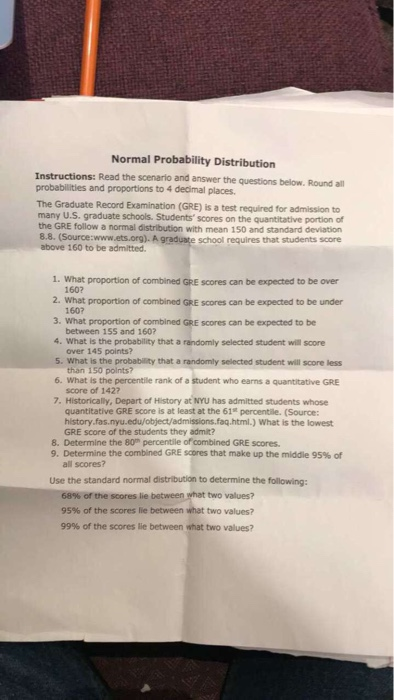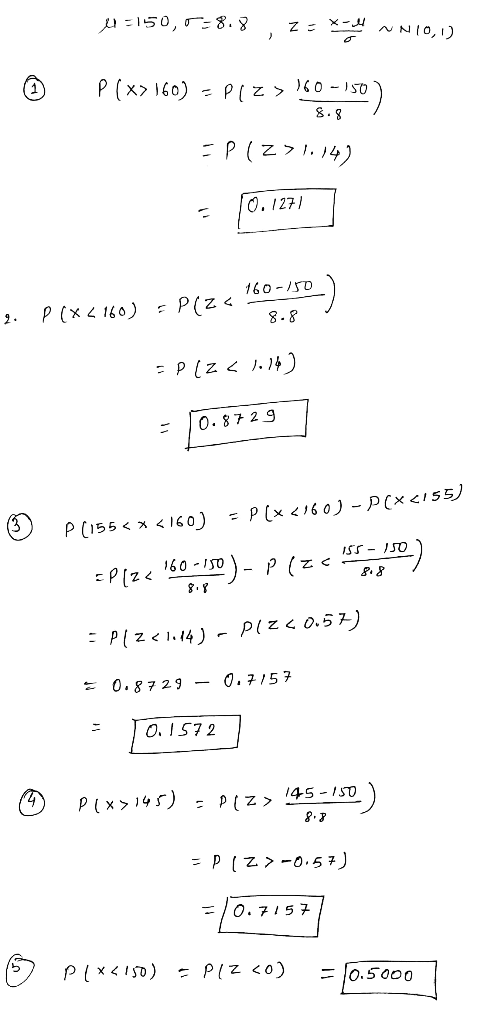#### Earn Coins

Coins can be redeemed for fabulous gifts.

Similar Homework Help Questions
• ### Instructions: Read the scenario and answer the questions below. Round all probabilities and proportions to 4...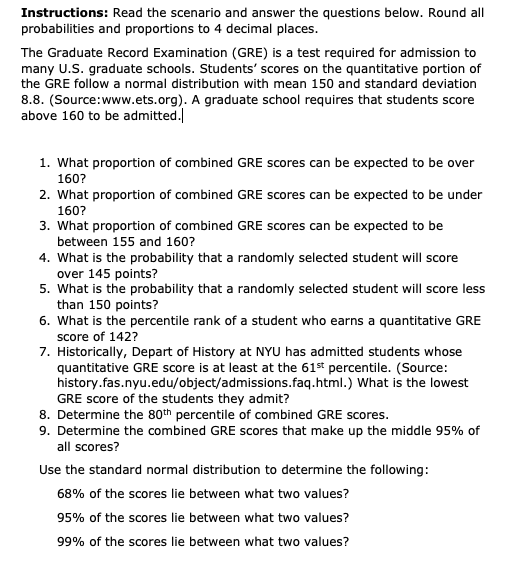Instructions: Read the scenario and answer the questions below. Round all probabilities and proportions to 4 decimal places. The Graduate Record Examination (GRE) is a test required for admission to many U.S. graduate schools. Students' scores on the quantitative portion of the GRE follow a normal distribution with mean 150 and standard deviation 8.8. (Source:www.ets.org). A graduate school requires that students score above 160 to be admitted. 1. What proportion of combined GRE scores can be expected to be over...

• ### To FOUR DECIMAL PLACES: Determine the area under the standard normal curve that lies to the...

To FOUR DECIMAL PLACES: Determine the area under the standard normal curve that lies to the left of Z = –1.31 to the right of Z = –2.47 between Z = –2.47 and Z = –1.31 between Z = 1.31 and Z = 2.47 Find the z-scores that separate the middle 84% of the standard normal distribution from the area in the tails. Find z0.18 a. Find the Z-score corresponding to the 72nd percentile. In other words, find the Z-score...

• ### Problem (ii): Cal Tech Engineering School only considers students in the top 12.5% based on their...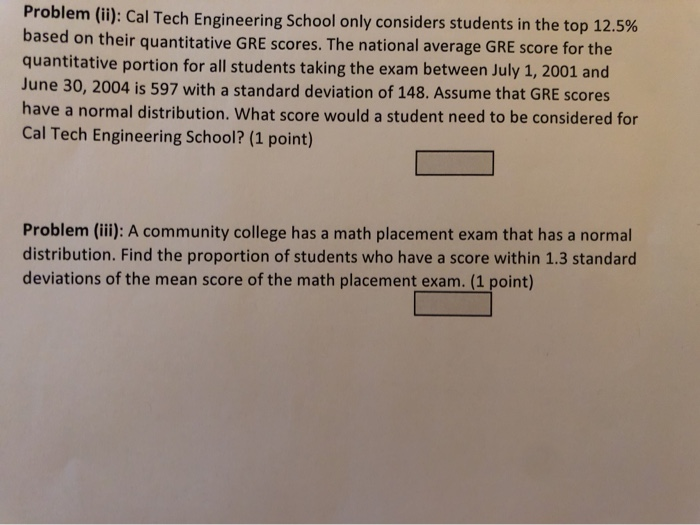Problem (ii): Cal Tech Engineering School only considers students in the top 12.5% based on their quantitative GRE scores. The national average GRE score for the quantitative portion for all students taking the exam between July 1, 2001 and June 30, 2004 is 597 with a standard deviation of 148. Assume that GRE scores have a normal distribution. What score would a student need to be considered for Cal Tech Engineering School? (1 point) Problem (iii): A community college has...

• ### 1. Suppose the proportion of the population in favor of a new law is 0.60. (a)...

1. Suppose the proportion of the population in favor of a new law is 0.60. (a) Suppose you take a sample of size n = 500. What is the probability that your sample proportion is 0.55 or lower? (b) Suppose that you take a sample of size n = 400 and find the sample proportion ˆp = 0.70. How many standard deviations is ˆp from the mean of the distribution of all sample proportions? 2. A college senior who took...

• ### Example 3 The scores on a midterm exam follow a normal distribution with an average of...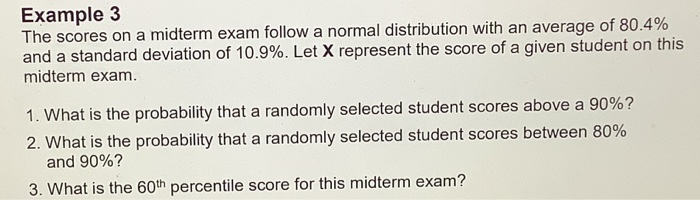Example 3 The scores on a midterm exam follow a normal distribution with an average of 80.4% and a standard deviation of 10.9%. Let X represent the score of a given student on this midterm exam. 1. What is the probability that a randomly selected student scores above a 90%? 2. What is the probability that a randomly selected student scores between 80% and 90%? 3. What is the 60th percentile score for this midterm exam?

• ### In a normal distribution of = 50 and SD = 7: What is the score at...

In a normal distribution of = 50 and SD = 7: What is the score at the 75th percentile (hint: on the table, find the z-score at .750 and change back to a raw score)? Find the percent of cases scoring above 55. Between what scores to the middle 25% of the cases lie? Beyond what scores do the most extreme cases lie?

• ### The scores on the SAT verbal test in recent years follow approximately the normal distribution distribution....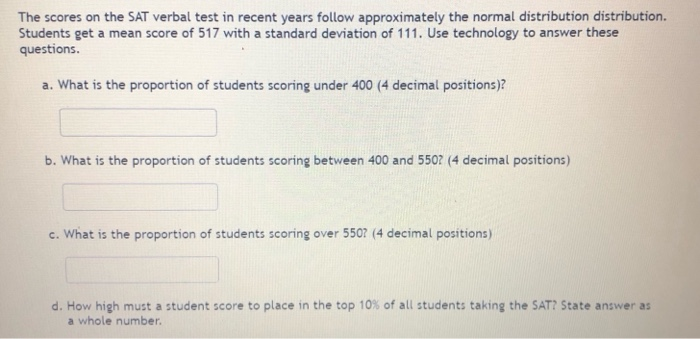The scores on the SAT verbal test in recent years follow approximately the normal distribution distribution. Students get a mean score of 517 with a standard deviation of 111. Use technology to answer these questions. a. What is the proportion of students scoring under 400 (4 decimal positions)? b. What is the proportion of students scoring between 400 and 5507 (4 decimal positions) c. What is the proportion of students scoring over 5507 (4 decimal positions) d. How high must...

• ### The scores on the SAT verbal test in recent years follow approximately the normal distribution distribution....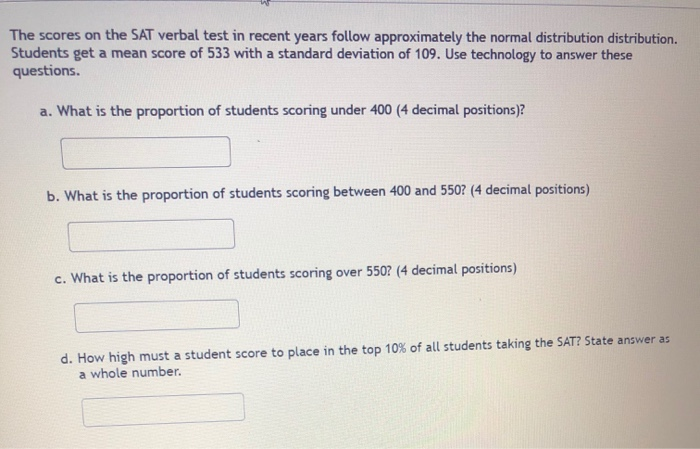The scores on the SAT verbal test in recent years follow approximately the normal distribution distribution. Students get a mean score of 533 with a standard deviation of 109. Use technology to answer these questions. a. What is the proportion of students scoring under 400 (4 decimal positions)? b. What is the proportion of students scoring between 400 and 550? (4 decimal positions) c. What is the proportion of students scoring over 550? (4 decimal positions) d. How high must...

• ### Assignment # 1 3: Between-Subjects ANOVA Instructions: For each research scenario, please complet...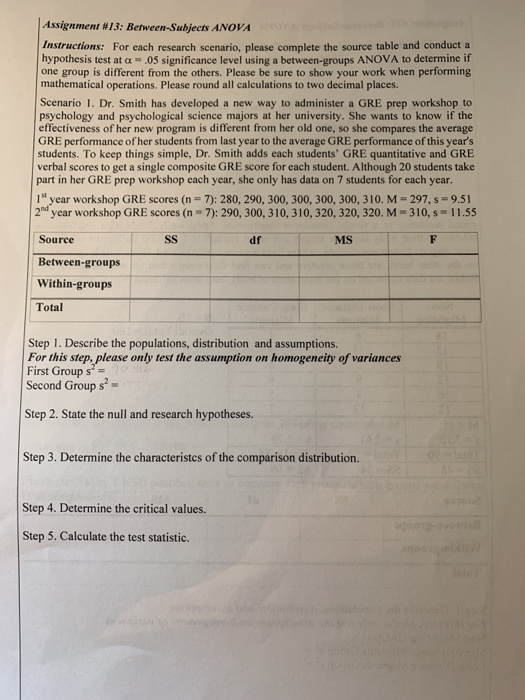please show all 6 steps with calculations! Assignment # 1 3: Between-Subjects ANOVA Instructions: For each research scenario, please complete the source table and conduct a hypothesis test at a-05 significance level using a between-groups ANOVA to determine if one group is different from the others. Please be sure to show your work when performing mathematical operations. Please round all calculations to two decimal places. Scenario 1. Dr. Smith has developed a new way to administer a GRE prep workshop...

• ### 1. Scores on a certain nationwide college entrance examination follow a normal distribution with a mean...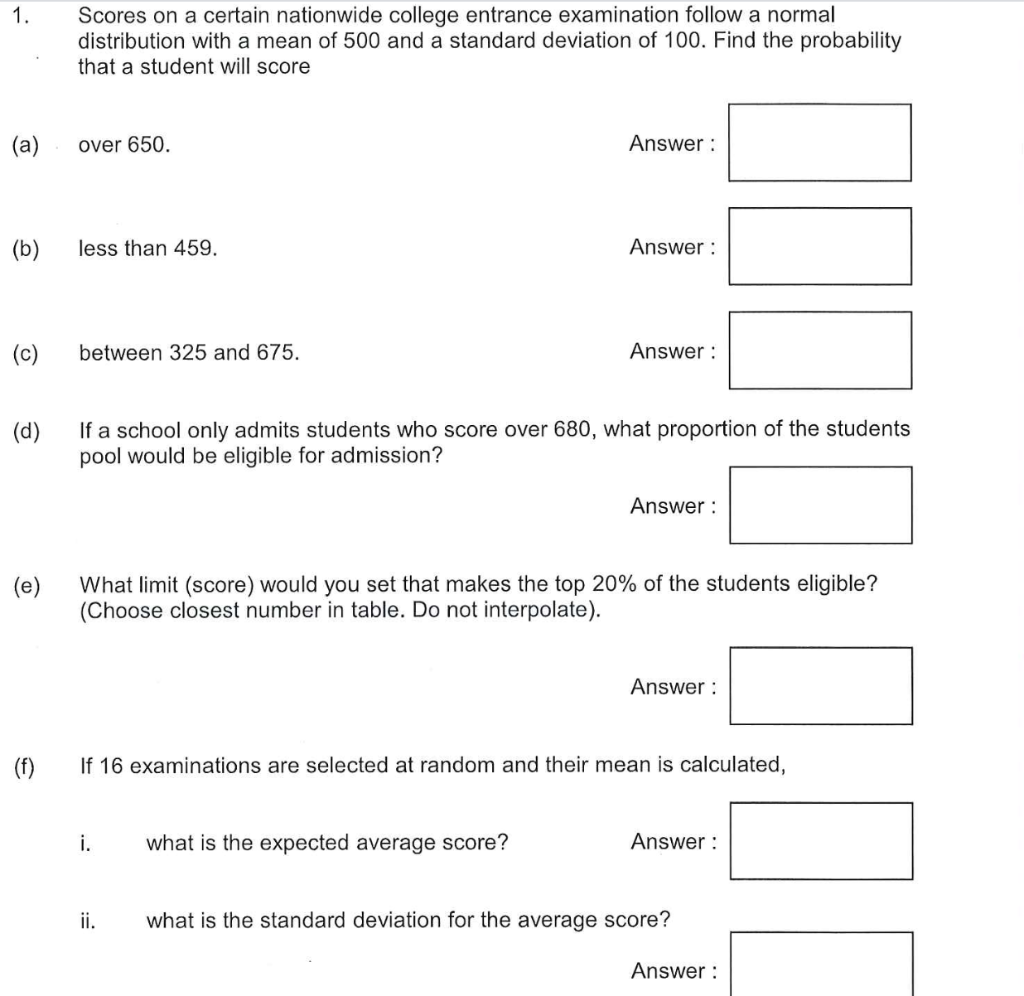1. Scores on a certain nationwide college entrance examination follow a normal distribution with a mean of 500 and a standard deviation of 100. Find the probability that a student will score (a) over 650. Answer: (b) less than 459. Answer: (C) between 325 and 675. Answer: (d) If a school only admits students who score over 680, what proportion of the students pool would be eligible for admission? Answer: (e) What limit (score) would you set that makes the...